« hover to pause »
« resume »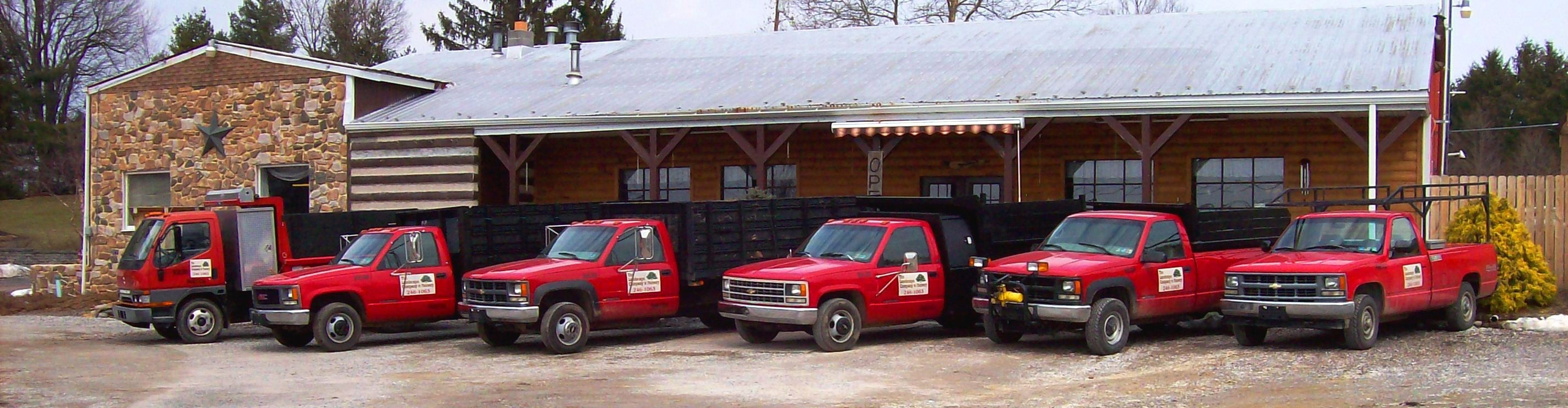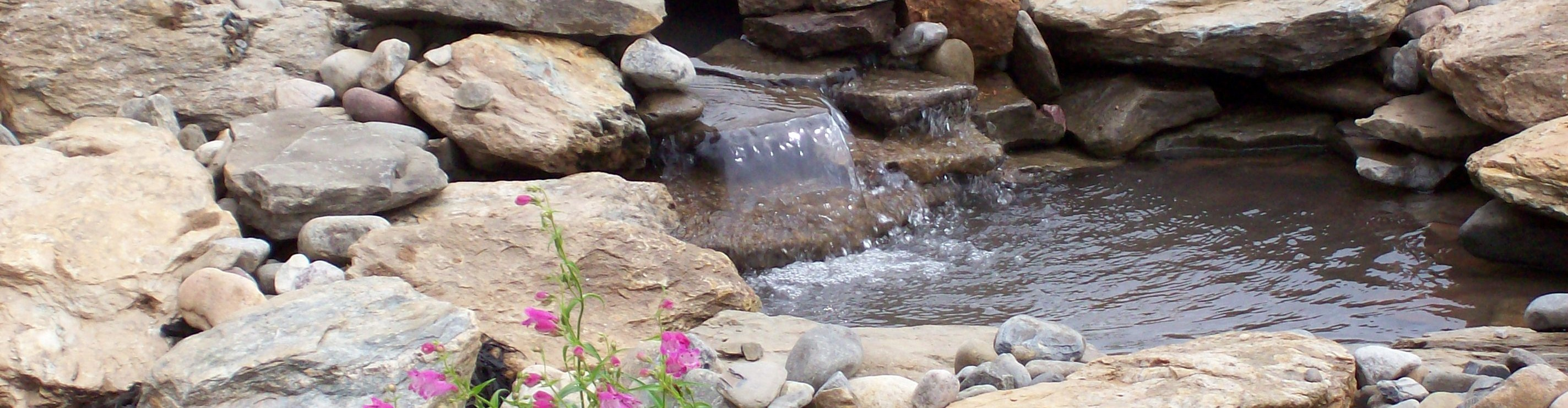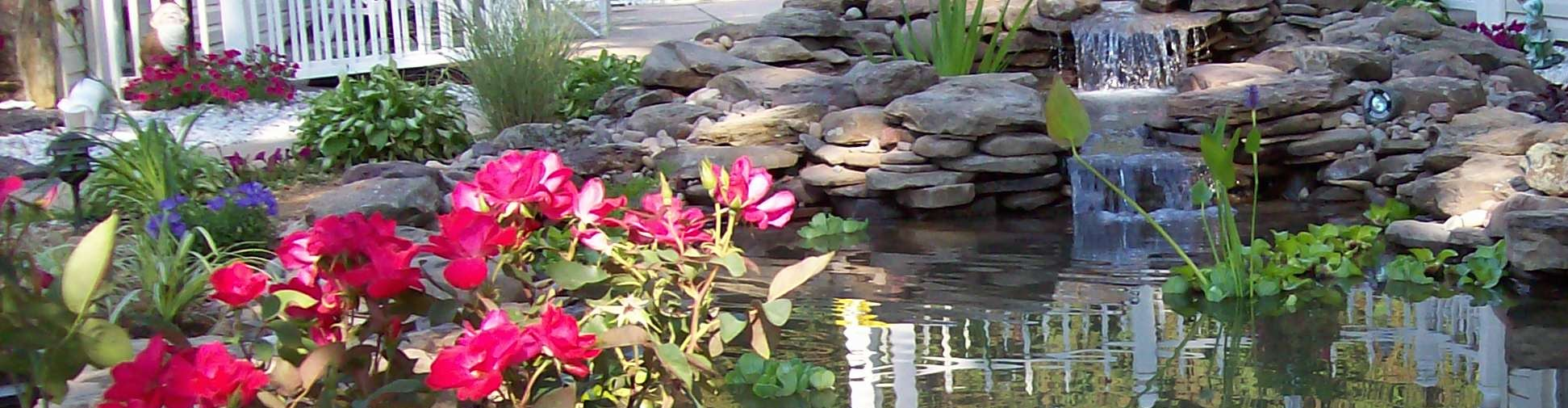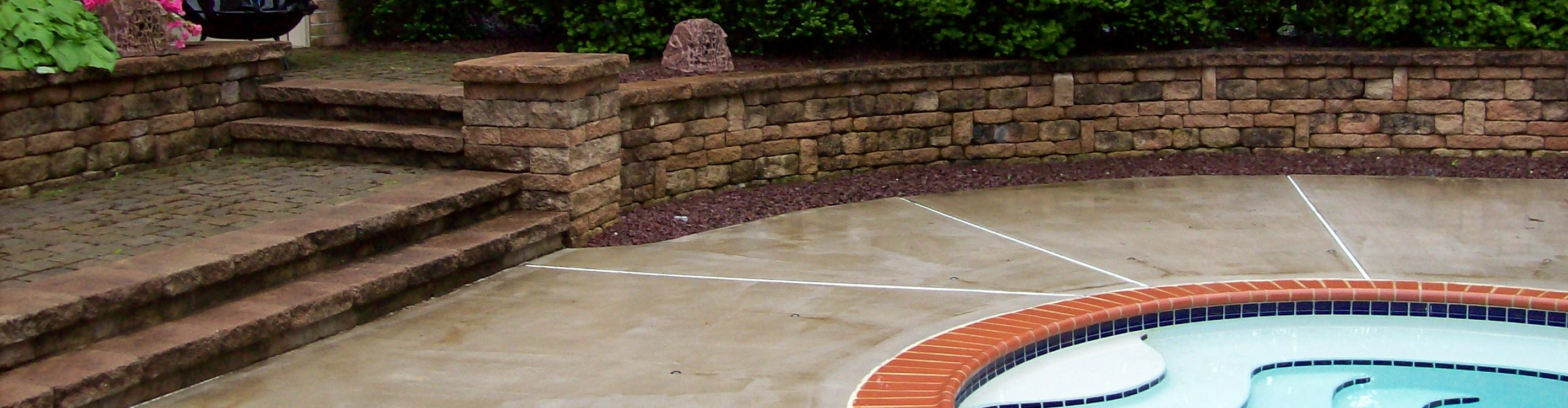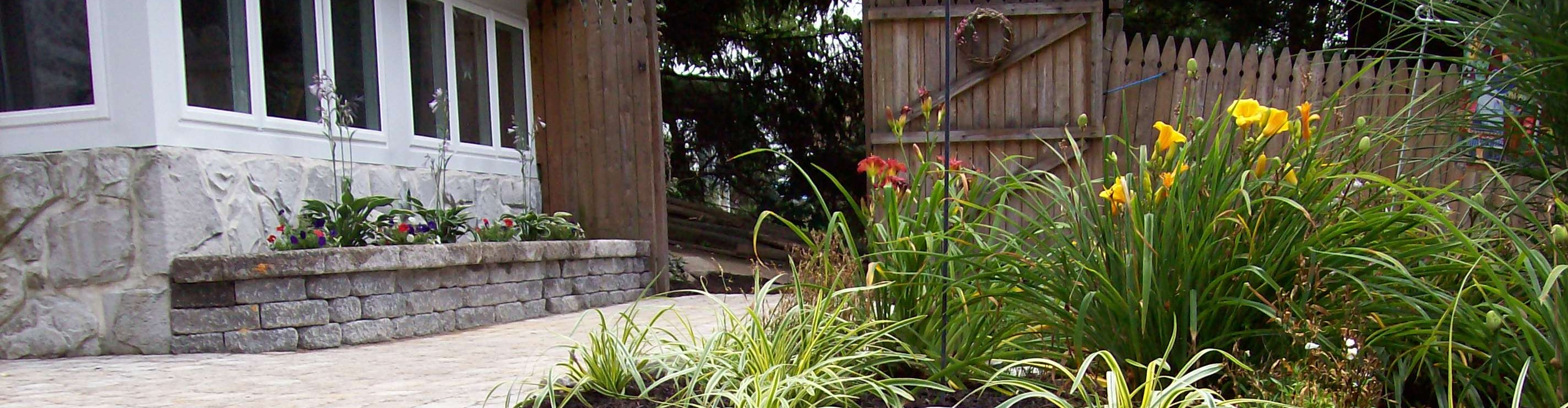## Product Coverage

How much mulch do I need?

1. First you need to figure out your square footage.  Multiply average length x average width.  (Ex. 8’x30′ = 240 Sq. Ft.)
2. Take the square footage and divide by 5.  (One bushel covers 5 square feet at a 2? depth.)  This number is the amount of bushels needed.  (Ex. 240 / 5 = 48 bushels)
3. Divide this number by 15.  (Our scoop equals 15 bushels.)  (Ex. 48 / 15 – 3.2 scoops)
4. 3.2 is the number of scoops required.  In most cases, you should round up to the next scoop.  In this case you should order 4 scoops.  That way you will have enough if you mulch more than 2? in some places.

Our scoop equals 2/3 cubic yard.

1 cubic yard = 22 bushels

1 cubic yard covers a 10′ x 10′ area at 2″ depth

Topsoil

1/2 yard covers 80 Sq. Ft. at a depth of 2″

1 scoop = approximately 1/2 cubic yard

What size pond liner do I need?

Width – Take width plus 2 x’s the depth and add at least one foot for overhang.

Length – Take length plus 2x’s the depth and add at least one foot for overhang.

We do not recommend seams!

Stone

Cubic Yards x 1.66 = Tons of stone needed

To figure cubic yards – multiply length x width x depth in feet.  This gives you cubic feet.  Then divide by 27 to get cubic yards.